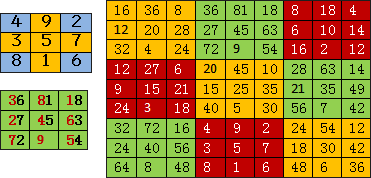《禹步九法》

 0 5 0 5 5 5 0 5 0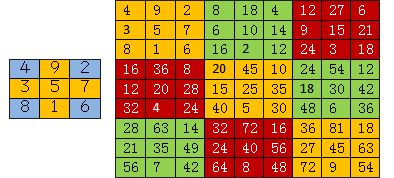(3×5)n n=1,2,3,4,5,6,7,8,9, 得，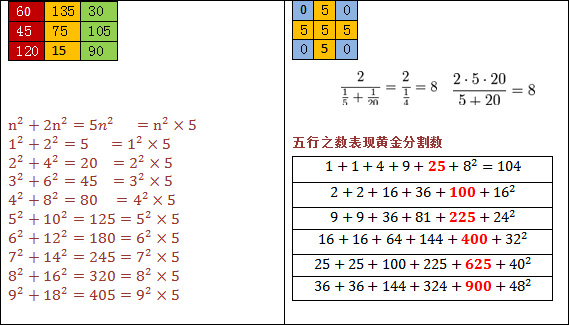2468四個偶數是0，我們把顏色也剪為兩色。會清晰地得到五行實四象虛的概念。這個概念在天文學中是最基本的天地陰陽法則的極概念數　。以此為本，合3,4,5,構造了四時，24節氣，72氣候。。。

 4+9+2 = 15 3+5+7 = 15 8+1+6 = 15 4+5+6 = 15 2+5+8 = 15 9+5+1 = 15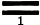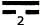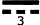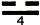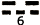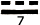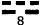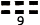1+0+9 = 10 2+0+8 = 10 3+0+7 = 10 4+0+6 = 10 5+0+5 = 10 0+5+0 = 5